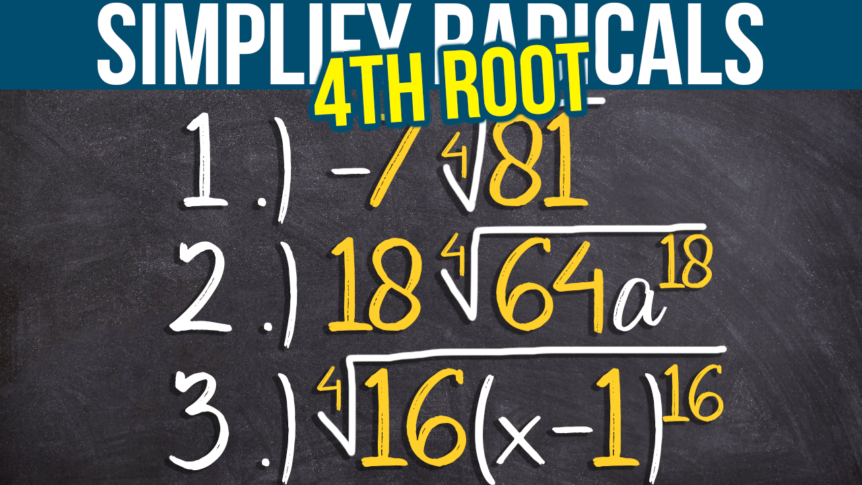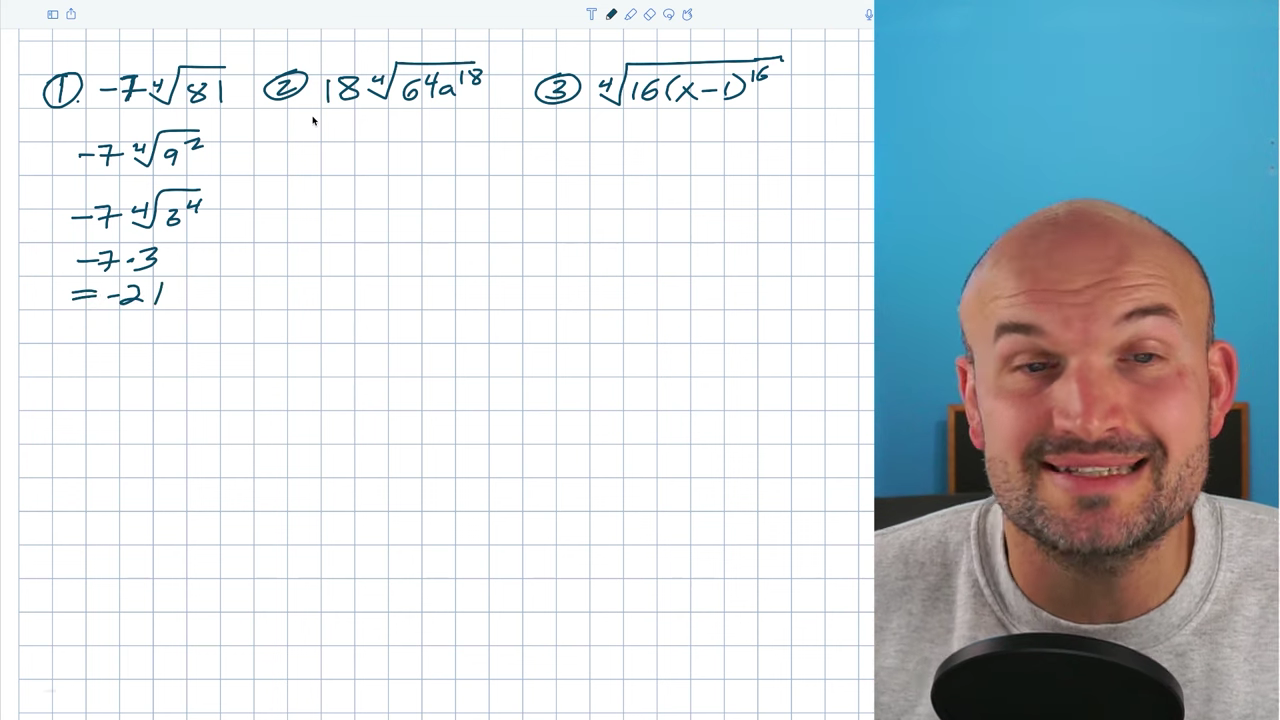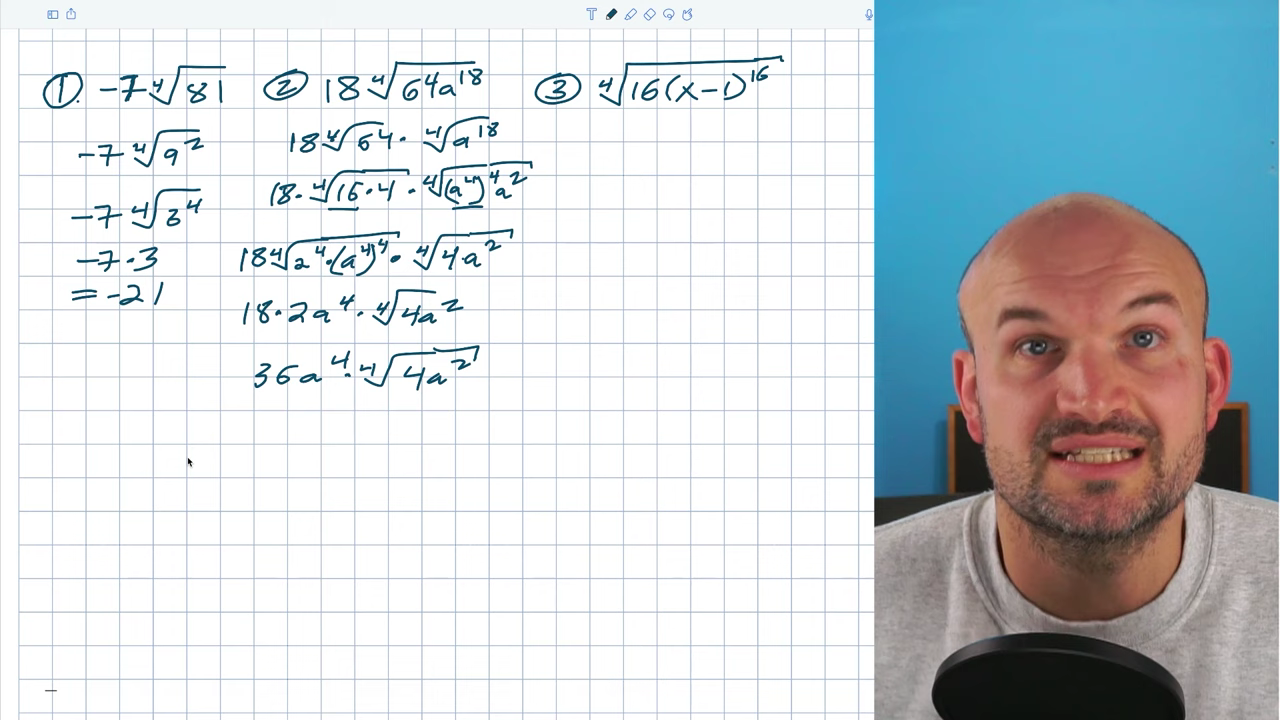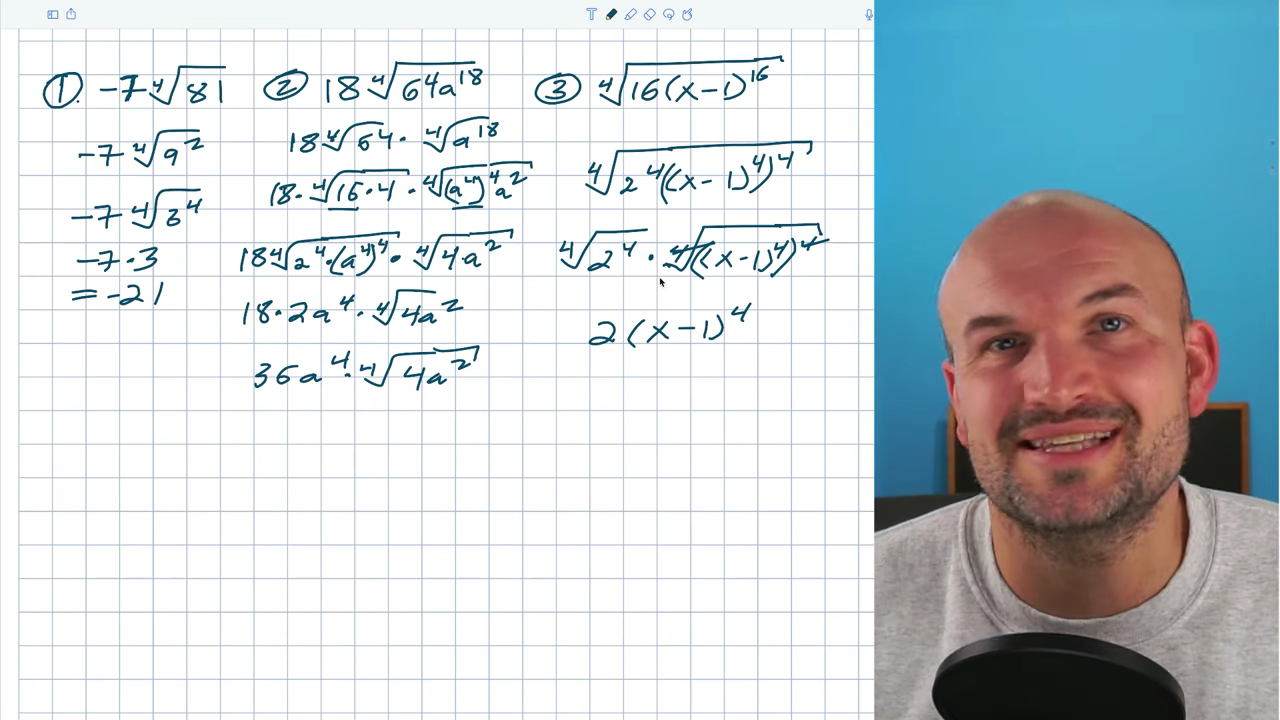# Simplifying the 4th Root: 3 Examples

I’m going to go over a few examples of simplifying the 4th root. Hopefully, my explanations will be helpful.

## Example #1: -7(4sqrt81)A lot of students get confused when they see something negative. You can’t take the square root, 4th root, or sixth root of a negative number, but because this negative is outside the radical, it won’t prevent us from taking the fourth root of what’s underneath it. When students see the 81, they’re often tempted to rewrite it as 9², but that doesn’t do anything for us. Our goal is to rewrite this as something raised to the fourth power, so we want to think about what number can be raised to the fourth power. The higher the index, the smaller the pool of numbers multiplied four times by themselves. 81 is what we call a quartic number because you can rewrite it as 3^4. Now, I have -7(4√3^4). I can take 4√3^4 and simplify it as 3, giving me -7⋅3 = -21.

## Example #2: 18(4sqrt64a^18)I will start by breaking up the throot of 64 and the fourth root of a^18. 18 is just multiplying on the outside, and our goal with the number is to find something being raised to the fourth power. 3^4 is 81, so the only thing that could make sense would be 2^4, which equals 16. 16 divides into 64, so I can simplify that. I want the fourth root of a^18 to be raised to the fourth power, so I’ll use the power rule. I have (a^4)^4, which gives me a^16. Then all I need to do is multiply by a² for a^18. I’ve now identified two values I can take: the fourth root of 16, which is 2, and the fourth root of (a^4)^4, which is a^4.

I’ll write 16 as 2^4. Now, I have 18⋅4√2^4(a^4)^4. I could separate this one more time, but hopefully, you recognize that I can take the fourth root of these separately, leaving me with 18⋅2a^4 ⋅ 4√4a², which I cannot simplify because they’re not raised to the fourth power. I can simplify further to 36a^4⋅4√4a².

## Example #3: 4sqrt16(x-1)^16Sometimes a problem has many moving parts, which can get confusing. What about when you have an expression, such as in this example? Don’t distribute the 16 to the (x-1) because it’s being raised to the 16th power. We to write the expression as being raised to the fourth power. I’ll rewrite 16 as (a^4)^4. Based on the power rule, 4⋅4 = 16. I can rewrite the first 16 as 2^4. Now, I have 2^4 and ((x-1)^4)^4, so I can take them from here. You could also separate them as 4√2^4 ⋅ 4√((x-1)^4)^4.

The fourth root of something raised to the fourth power is 2, and even though both parts of the second part are raised to the fourth, we can only take the inverse operation of the top expression. My final answer is 2(x-1)^4. Hopefully, your teacher will let you leave your answer like that.

I hope this was helpful for you. If so, consider subscribing to my YouTube channel for more content like this. Comment below if you have any questions, or check out more examples I’ve linked below.

Simplify the Square Root – https://youtu.be/XQCWSqNN69Q

Simplify the 3rd Root – https://youtu.be/HVkhCs3Qr8Y